Next: Scalar Product Up: Vector Algebra and Vector Previous: Cartesian Components of a

# Coordinate Transformations

A Cartesian coordinate system allows position and direction in space to be represented in a very convenient manner. Unfortunately, such a coordinate system also introduces arbitrary elements into our analysis. After all, two independent observers might well choose coordinate systems with different origins, and different orientations of the coordinate axes. In general, a given vector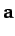will have different sets of components in these two coordinate systems. However, the direction and magnitude ofare the same in both cases. Hence, the two sets of components must be related to one another in a very particular fashion. Actually, since vectors are represented by moveable line elements in space (i.e., in Figure A.97,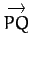and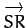represent the same vector), it follows that the components of a general vector are not affected by a simple shift in the origin of a Cartesian coordinate system. On the other hand, the components are modified when the coordinate axes are rotated.

Suppose that we transform to a new coordinate system,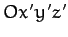, which has the same origin as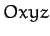, and is obtained by rotating the coordinate axes ofthrough an angle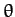about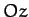--see Figure A.100. Let the coordinates of a general point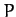beinand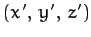in. According to simple trigonometry, these two sets of coordinates are related to one another via the transformation: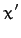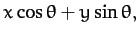(1277)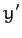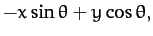(1278)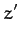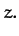(1279)

Consider the vector displacement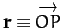. Note that this displacement is represented by the same symbol,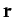, in both coordinate systems, since the magnitude and direction ofare manifestly independent of the orientation of the coordinate axes. The coordinates ofdo depend on the orientation of the axes: i.e.,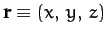in, and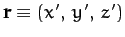in. However, they must depend in a very specific manner [i.e., Equations (A.1277)-(A.1279)] which preserves the magnitude and direction of.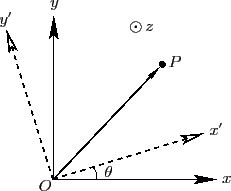The components of a general vectortransform in an analogous manner to Equations (A.1277)-(A.1279): i.e.,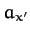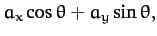(1280)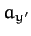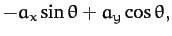(1281)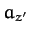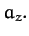(1282)

Moreover, there are similar transformation rules for rotation about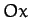and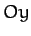. Equations (A.1280)-(A.1282) effectively constitute the definition of a vector: i.e., the three quantities (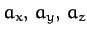) are the components of a vector provided that they transform under rotation of the coordinate axes aboutin accordance with Equations (A.1280)-(A.1282). (And also transform correctly under rotation aboutand). Conversely, () cannot be the components of a vector if they do not transform in accordance with Equations (A.1280)-(A.1282). Of course, scalar quantities are invariant under rotation of the coordinate axes. Thus, the individual components of a vector (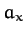, say) are real numbers, but they are not scalars. Displacement vectors, and all vectors derived from displacements (e.g., velocity, acceleration), automatically satisfy Equations (A.1280)-(A.1282). There are, however, other physical quantities which have both magnitude and direction, but which are not obviously related to displacements. We need to check carefully to see whether these quantities are really vectors (see Section A.8).Next: Scalar Product Up: Vector Algebra and Vector Previous: Cartesian Components of a
Richard Fitzpatrick 2011-03-31